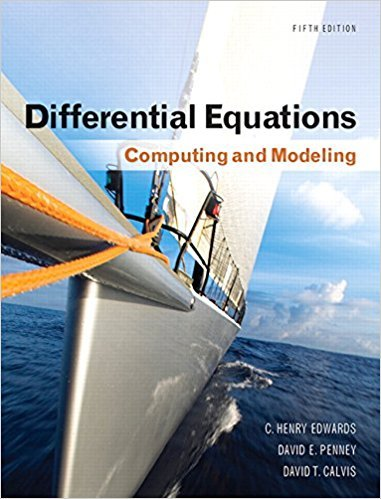×
×

# Solutions for Chapter 4: Introduction to Systems of Differential Equations## Full solutions for Differential Equations: Computing and Modeling | 5th Edition

ISBN: 9780321816252Solutions for Chapter 4: Introduction to Systems of Differential Equations

Solutions for Chapter 4
4 5 0 359 Reviews
19
1
##### ISBN: 9780321816252

This textbook survival guide was created for the textbook: Differential Equations: Computing and Modeling, edition: 5. This expansive textbook survival guide covers the following chapters and their solutions. Chapter 4: Introduction to Systems of Differential Equations includes 48 full step-by-step solutions. Differential Equations: Computing and Modeling was written by and is associated to the ISBN: 9780321816252. Since 48 problems in chapter 4: Introduction to Systems of Differential Equations have been answered, more than 1909 students have viewed full step-by-step solutions from this chapter.

Key Math Terms and definitions covered in this textbook
• Affine transformation

Tv = Av + Vo = linear transformation plus shift.

• Complex conjugate

z = a - ib for any complex number z = a + ib. Then zz = Iz12.

• Ellipse (or ellipsoid) x T Ax = 1.

A must be positive definite; the axes of the ellipse are eigenvectors of A, with lengths 1/.JI. (For IIx II = 1 the vectors y = Ax lie on the ellipse IIA-1 yll2 = Y T(AAT)-1 Y = 1 displayed by eigshow; axis lengths ad

• Free columns of A.

Columns without pivots; these are combinations of earlier columns.

• Free variable Xi.

Column i has no pivot in elimination. We can give the n - r free variables any values, then Ax = b determines the r pivot variables (if solvable!).

• Gauss-Jordan method.

Invert A by row operations on [A I] to reach [I A-I].

• Hankel matrix H.

Constant along each antidiagonal; hij depends on i + j.

• Hilbert matrix hilb(n).

Entries HU = 1/(i + j -1) = Jd X i- 1 xj-1dx. Positive definite but extremely small Amin and large condition number: H is ill-conditioned.

• Identity matrix I (or In).

Diagonal entries = 1, off-diagonal entries = 0.

• Left inverse A+.

If A has full column rank n, then A+ = (AT A)-I AT has A+ A = In.

• Matrix multiplication AB.

The i, j entry of AB is (row i of A)·(column j of B) = L aikbkj. By columns: Column j of AB = A times column j of B. By rows: row i of A multiplies B. Columns times rows: AB = sum of (column k)(row k). All these equivalent definitions come from the rule that A B times x equals A times B x .

• Multiplication Ax

= Xl (column 1) + ... + xn(column n) = combination of columns.

• Normal matrix.

If N NT = NT N, then N has orthonormal (complex) eigenvectors.

• Nullspace N (A)

= All solutions to Ax = O. Dimension n - r = (# columns) - rank.

• Outer product uv T

= column times row = rank one matrix.

• Permutation matrix P.

There are n! orders of 1, ... , n. The n! P 's have the rows of I in those orders. P A puts the rows of A in the same order. P is even or odd (det P = 1 or -1) based on the number of row exchanges to reach I.

• Pivot.

The diagonal entry (first nonzero) at the time when a row is used in elimination.

• Reduced row echelon form R = rref(A).

Pivots = 1; zeros above and below pivots; the r nonzero rows of R give a basis for the row space of A.

• Tridiagonal matrix T: tij = 0 if Ii - j I > 1.

T- 1 has rank 1 above and below diagonal.

• Volume of box.

The rows (or the columns) of A generate a box with volume I det(A) I.

×